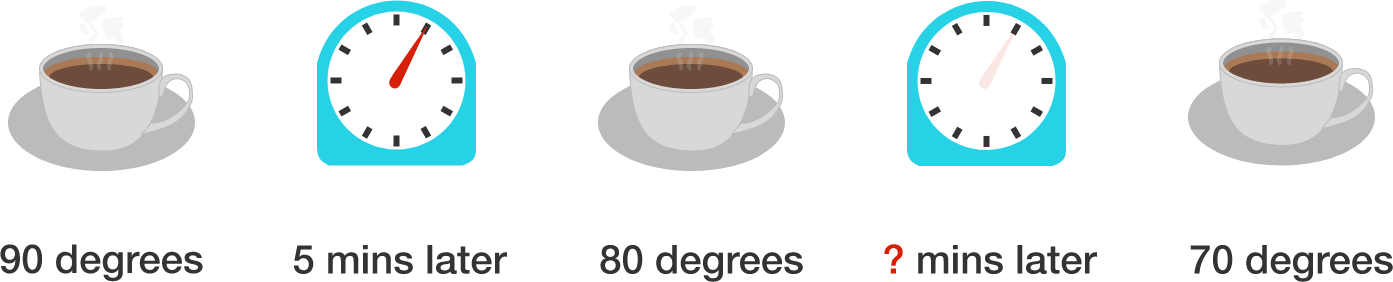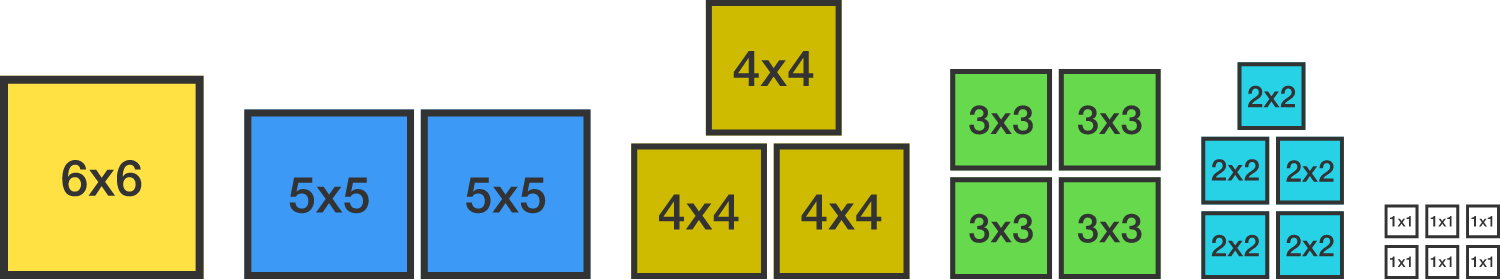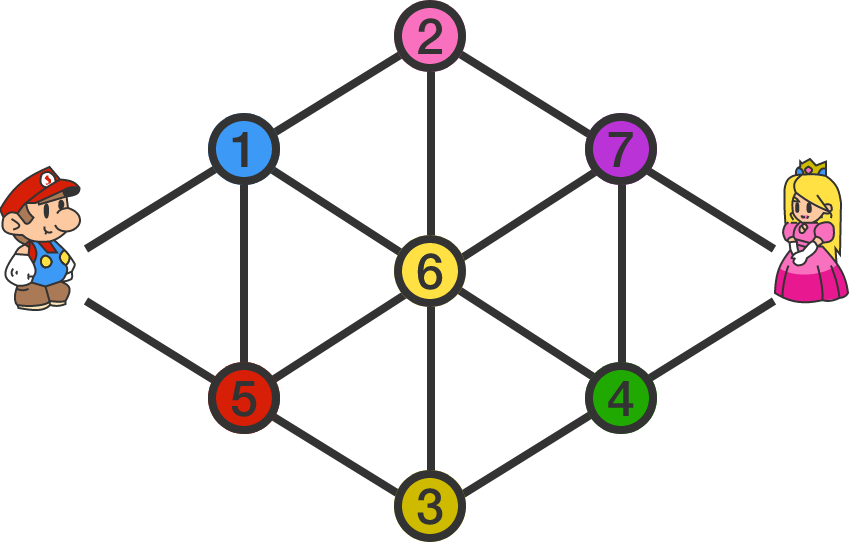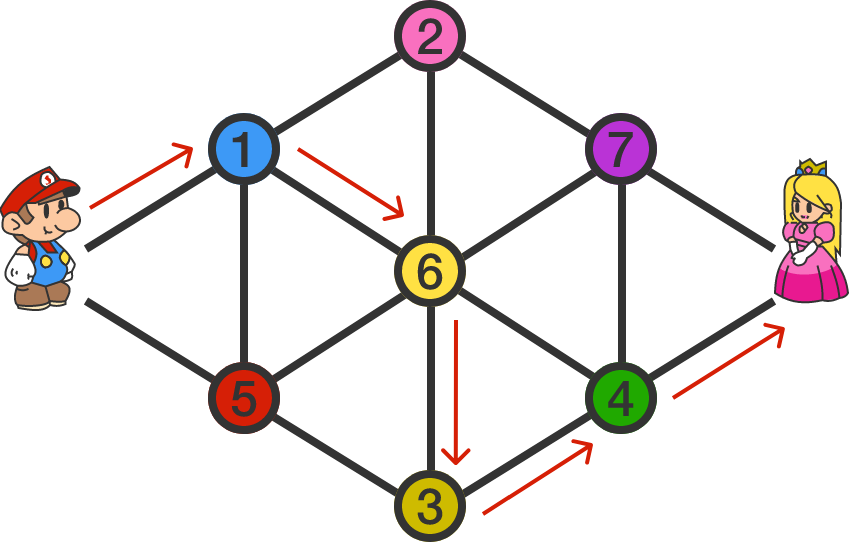# Problems of the Week

Contribute a problem

# 2017-02-20 Basic

What is the value of $\dfrac{11!-10!}{9!}?$


Notation: $!$ is the factorial notation. For example, $8! = 1\times2\times3\times\cdots\times8$.John makes a cup of coffee, which is initially at a temperature of $90^\circ\text{C}$. After 5 minutes, he notices that its temperature has dropped to $80^\circ \text{C}$. How much more time will it take for the temperature of the coffee to further drop to $70^\circ \text{C}$?Can you use all of the pieces to build a large square? And if possible, what is its side length?

• one $6 \times 6$ square
• two $5 \times 5$ squares
• three $4 \times 4$ squares
• four $3 \times 3$ squares
• five $2 \times 2$ squares
• six $1 \times 1$ squares

$\large \begin{array} { l l l l l } & &D & O & G\\ +& &C & A & T \\ \hline & 1 & 0 & 0 & 0 \\ \end{array}$

The above shows a long addition, where each of the symbols represents a distinct single-digit positive integer.

What is the sum $D + O + G + C + A + T ?$Mario has to meet the Princess at the opposite node of the network, as shown above. The digit on each node indicates the number of coins he collects upon arriving at the node. He can visit each node only once.

For example, if Mario follows the red arrow trail illustrated below, he will obtain $1+6+3+4=14$ coins before reaching the Princess.To prove his love, the Princess demands that Mario collect exactly 17 coins (as that is her favorite number). Can Mario accomplish this task?

×